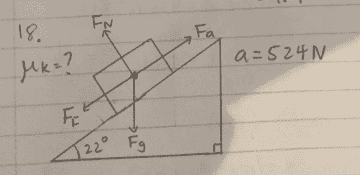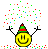# Coefficient of kinetic friction

• DonutHole
I see so if that isn't the problem what do...In summary, the equation for calculating the friction force between two objects is Fa - mg sinθ + μmg cos θ. Newton's second law states that the external force applied should equal ma. When friction is present, the force of friction is downward, towards the bottom of the slope.

#### DonutHole

Homework Statement
You are pushing a 53kg crate with an acceleration of 1m/s^2 up a ramp. The ramp makes an angle of 22° above the horizontal. If your applied force is 524N, what is the kinetic coefficient of friction between the crate and the ramp?
Relevant Equations
μ = Ff/Fn
Fn = m X g X cos θ
Fnet = Fa – mg sin θ +or- μmg cos θ
Fn = 53 X 9.81 X cos 22
Fnet = 524 – 53 X 9.81 sin 22 + μ X 53 X 9.81 cos 22Is that all you've got? What about the solution?

PeroK said:Is that all you've got? What about the solution?
I am not sure how to incorporate the acceleration of 1m/s^2 into the equation.

DonutHole said:
I am not sure how to incorporate the acceleration of 1m/s^2 into the equation.
DonutHole said:
Fnet = Fa – mg sin θ +or- μmg cos θ
That equation looks good, although you'll have to decide whether it should be ##+## or ##-##. And shouldn't that just be ##F##? What's the ##a## in ##Fa##?

What about Newton's second law? Isn't that relevant?

•Chestermiller
PeroK said:
That equation looks good, although you'll have to decide whether it should be ##+## or ##-##. And shouldn't that just be ##F##? What's the ##a## in ##Fa##?

What about Newton's second law? Isn't that relevant?
I think the equation should be Fa - mg sinθ + μmg cos θ
a in Fa is the acceleration
and Newtons 2nd law says that the external forces applied should equal ma which means that all the components of the equation should equal mass times acceleration?

DonutHole said:
I think the equation should be Fa - mg sinθ + μmg cos θ
a in Fa is the acceleration
I don't think I've ever seen ##Fa## in an equation. The dimensions are wrong, as what you have is an equation between forces. You can't have ##Fa## in there.

By having ##+\mu mg \cos \theta## you have friction acting up the slope. Does that look right?

DonutHole said:
and Newtons 2nd law says that the external forces applied should equal ma which means that all the components of the equation should equal mass times acceleration?
Yes, that's a second equation: ##F_{net} = ma##.

PeroK said:
By having ##+\mu mg \cos \theta## you have friction acting up the slope. Does that look right.
shouldn't friction be acting towards the bottom of the slope because applied force is acting towards the top?

DonutHole said:
shouldn't friction be acting towards the bottom of the slope because applied force is acting towards the top?
In this case, yes, friction is acting down the slope, because the applied force is large enough to accelerate the block up the slope. If the applied force were less, then the block might be trying to slide down the slope with friction opposing the motion.

PeroK said:
In this case, yes, friction is acting down the slope, because the applied force is large enough to accelerate the block up the slope. If the applied force were less, then the block might be trying to slide down the slope with friction opposing the motion.
This is my first time doing a question like this so I am not too sure but the equation ma = F - mg sinθ + μmg cos θ is correct? and if that's the case what is F?

DonutHole said:
This is my first time doing a question like this so I am not too sure but the equation ma = F - mg sinθ + μmg cos θ is correct? and if that's the case what is F?
##F## is the applied force. Note that I guess we should assume that it acts parallel to the ramp.

I'm still not convinced by the sign of that force of friction!

PeroK said:
##F## is the applied force. Note that I guess we should assume that it acts parallel to the ramp.

I'm still not convinced by the sign of that force of friction!
Yes the applied force is parallel to the ramp.I should have shared this free body diagram earlier

DonutHole said:
Yes the applied force is parallel to the ramp. View attachment 291503
I should have shared this free body diagram earlier
Okay, apart from having ##Fa## rather than simply ##F## that looks good. Can you finish off this problem? You seem to be nearly there.

PeroK said:
Okay, apart from having ##Fa## rather than simply ##F## that looks good. Can you finish off this problem? You seem to be nearly there.
the answer and step I got are:

ma = F - mg sinθ + μmg cos θ
53(1) = 524 - (53)(9.81)sin22 + μ(53)(9.81)cos22
53 = 329 + 482μ
μ = -0.57

is this correct?

DonutHole said:
the answer and step I got are:

ma = F - mg sinθ + μmg cos θ
53(1) = 524 - (53)(9.81)sin22 + μ(53)(9.81)cos22
53 = 329 + 482μ
μ = -0.57

is this correct?
I've never heard of a negative coefficient of friction! Maybe you got the sign of the friction force wrong?

PeroK said:
I've never heard of a negative coefficient of friction! Maybe you got the sign of the friction force wrong?
that's what i thought too maybe it should have been 524 + the rest instead of -?

DonutHole said:
that's what i thought too maybe it should have been 524 + the rest instead of -?
I would vote for ##-##.

PeroK said:
I would vote for ##-##.
I see so if that isn't the problem what do you think I got wrong?

Plus = up the slope and minus = down the slope.

Your error is to have friction acting up the slope. E.g.

DonutHole said:
ma = F - mg sinθ + μmg cos θ

PeroK said:
Plus = up the slope and minus = down the slope.

Your error is to have friction acting up the slope. E.g.
so the equation would be ma = F - mg sinθ - μmg cos θ

•PeroK
DonutHole said:
so the equation would be ma = F - mg sinθ - μmg cos θPeroK said:in that case the answer would be 0.57

•PeroK